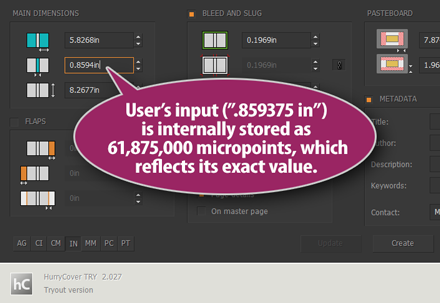Interesting question! As you probably have observed, InDesign itself does not display all entered digits in the Transform panel. If you set a rectangle to be .859375 inches wide, what you get in the GUI is `0.8594 in`. As far as I know InDesign's decimal precision is 1/10,000 (up to 4 decimal points) and users have no control over this.

At a lower technical level, all measurements in InDesign are coerced into PostScript points with a decimal precision which can't be higher than 7 digits since the underlying code (cf. InDesign's SDK) uses a machine epsilon of `1e-8`, as shown in the header `PMReal.h`. As Adobe developers comment, the class `PMReal` is used, instead of double, “primarily to hide the epsilon comparisons behind operator definitions.” This also means that “implicit conversion happens when going to a PMReal”.

Going back to InDesign measurement units seen from the scripting layer, many experiments have shown that a machine epsilon is required as well in ExtendScript, in order to compare object locations, geometric bounds, and so on. There are two main difficulties: on the one hand we can't tell how precisely JavaScript numbers back-and-forth interact with the subsystem, and on the other hand we don't know when unit conversions actually occur. In addition, floating point arithmetic based on IEEE754 specification—either 32 or 64 bits, depending on the operating system—has severe limitations in representing reliable decimal values.

For all these reasons I strongly (although empirically) suspect that InDesign can't actually handle a precision deeper than `.001 pt` (that is, `.0003528 mm`, or `.3528 µm`.) Since one international inch is 72 (PostScript) points, a precision of `.0001 inches` is equivalent to `.0072 pt`, that is `2.54016 µm`. Note that a red blood cell is about 7 µm. So, in the considered example (`".859375 in"`) the last two digits likely have the order of magnitude of the cell membrane thickness.

## How Does HurryCover Handle Those Decimals?

Anyway, HurryCover tries to deal at best as it can with precise measurements. Actually, all values are internally stored as integers in a custom micropoint unit. In this unit, `1` represents one millionth of a PostScript point. The highest value that HurryCover allows (maximum height) is 3,685,000,000 micropoints (about 1.3 m.) Since ExtendScript can safely handle signed integers in the range `)-2^53,+2^53(`, HurryCover's internal unit avoids many floating point arithmetic issues.

What the user sees, however, is a rounded expression of the underlying numbers. When the [PT] unit is in use values are shown with 2 decimal digits. When [IN] is used, up to 4 decimal digits are displayed. In either case, the value you entered is converted into, and stored as, a micropoint number. For example, setting manually the spine to `".859375 in"` results in storing the number 61,875,000 (micropoints.) As you can check this exactly reflects the original input, since `61.875 / 72 = 0.859375`.So, although HurryCover displays `"0.8594in"` in the main dialog, it actually sends the value 61.875 pt to InDesign in that specific case. From then, we can't know what kind of approximations the application will apply. (As said above I do not bet on an accuracy greater than .001 pt.)

Note. — Incidentally, it should also be pointed out that HurryCover's precision may be impacted by multiple unit conversions. Indeed, if the user switches from [IN] to [PT], then edit some fields, then goes back to [IN], the script has to decide whether the internal values need to be changed with respect to the edited values.